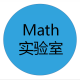# 标题

Autem vel eum iriure dolor in hendrerit in vulputate velit esse molestie consequat, vel illum dolore eu feugiat nulla facilisis at vero eros et dolore feugait

# 中考总复习：数与式专题讲义—整式的考点知识梳理分析说明

 考点 知识点分析 考查星级 整式的 相关概念 1．单项式：数字或字母的乘积叫做单项式，所有字母的指数的和叫做单项式的次数；单独的一个数或一个字母也是单项式； 2．多项式：几个单项式的和叫做多项式，每个单项式叫做多项式的项，不含字母的项是常数项，次数最高的项的次数是多项式的次数； 3．($$1$$)同类项：所含字母相同，相同字母的指数也相同的项叫做同类项； ($$2$$)合并同类项：把系数相加，所含字母及字母的指数不变； 4．去括号：去括号时，括号前面是“$$+$$”时，去掉括号后，括号里的项都不改变符号，括号前面是“$$-$$”时，去掉括号后，括号里的项改变符号； 如：$$+(a-b)-=a-b$$，$$-(a-b)=-a+b$$； ★☆☆☆☆​ 整式的运算及求值 1．整式的加减：实质是合并同类项，若有括号，先去括号，再合并同类项； 2．整式乘法 ($$1$$)幂的运算： ①$$a^m·a^n=a^{m+n}$$($$m$$，$$n$$为正整数，$$a≠0$$)； ②$$(a^m)^n=a^{mn}$$($$m$$，$$n$$为正整数，$$a≠0$$)． ③$$(ab)^n=a^nb^n$$($$m$$，$$n$$为正整数，$$a≠0$$)． ④$$a^m÷a^n=a^{m-n}$$($$m$$，$$n$$为正整数，$$m>n$$，$$a≠0$$)． ($$2$$)整式乘法：$$(a+b)(c+d)=ac+ad+bc+bd$$； ($$3$$)乘法公式： ①平方差公式：$$(a+b)(a-b)=a^2-b^2$$； ②完全平方公式：$$(a±b)^2=a^2±2ab+b^2$$； 3．整式除法：单项式除以单项式，把系数、相同字母的幂分别相除，作为商的因式，只在被除式中含有的字母，直接作为商的因式；多项式除以单项式，用多项式的每一个项分别除以单项式，再把所得的商相加． ★★★★☆​ 因式分解 1．定义：把一个多项式化成几个整式乘积的形式； 2．常用方法： ($$1$$)提公因式法； ($$2$$)公式法； ($$3$$)分组分解法； ($$4$$)十字相乘法； ★★★☆☆​​

2019.5.16Math实验室教研资料交流群：764502354333 views